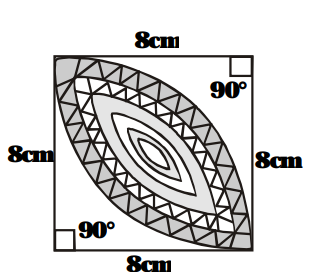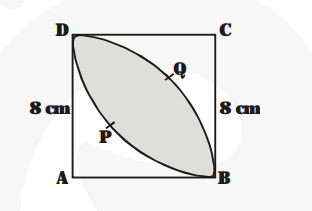# Calculate the area of the designed region in fig.

Question:

Calculate the area of the designed region in fig. common between the two quadrants of circles of radius 8 cm each.Solution:

Side of the square = 8 cm

$\therefore$ Area of the square $(\mathrm{ABCD})=8 \times 8 \mathrm{~cm}^{2}$

$=64 \mathrm{~cm}^{2}$

$\therefore \quad$ Area of the quadrant $\mathrm{ADQB}$

$=\frac{90^{\circ}}{360^{\circ}} \times \frac{22}{7} \times 8 \times 8 c m^{2}$

$=\frac{1}{4} \times \frac{22}{7} \times 64 \mathrm{~cm}^{2}=\frac{22 \times 16}{7} \mathrm{~cm}^{2}$

$\mathrm{BPDC}=\frac{\mathbf{2 2} \times \mathbf{1 6}}{\mathbf{7}} \mathrm{cm}^{2}$$=2\left[\frac{22 \times 16}{7}\right] \mathrm{cm}^{2}=\frac{704}{7} \mathrm{~cm}^{2}$

Now, area of design =

[Sum of the area of two quadrant] –

[Area of the square ABCD]

$=\frac{704}{7} \mathrm{~cm}^{2}-64 \mathrm{~cm}^{2}=\frac{704-448}{7} \mathrm{~cm}^{2}$

$=\frac{\mathbf{2 5 6}}{\mathbf{7}} \mathrm{cm}^{2}$